# (GENERAL INTELLIGENCE AND REASONING)

QID : 201 -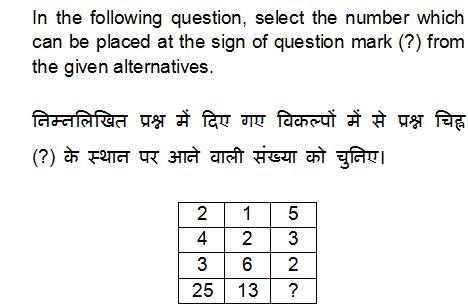1) 16
2) 30
3) 31
4) 41
QID : 202 -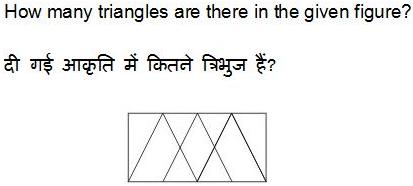1) 8
2) 10
3) 12
4) 9
QID : 203 -1) 00, 11, 32, 56, 76
2) 95, 67, 32, 56, 12
3) 00, 67, 78, 04, 76
4) 95, 11, 33, 55, 77
Correct Answer: 00, 67, 78, 04, 76
QID : 204 -1)2)3)4)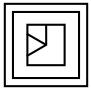QID : 205 -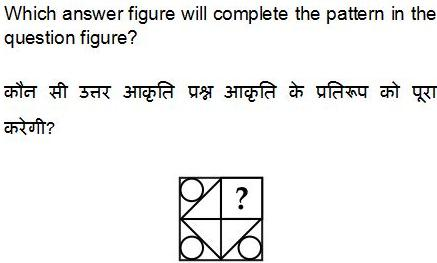1)2)3)4)QID : 206 -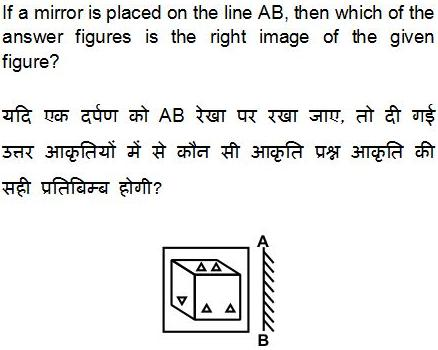1)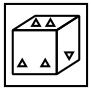2)3)4)QID : 207 -1)2)3)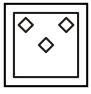4)QID : 208 - If ‘A’ means ‘+’, ‘B’ means ‘–’, ‘C’ means ‘×’ and ‘D’ means ‘÷’, then which of the following equation is true?
1) 12 D 6 A 4 C 3 = 12
2) 48 D 3 C 2 = 31
3) 14 C 2 D 7 A 3 = 6
4) 24 C 3 B 14 = 58
Correct Answer: 24 C 3 B 14 = 58
QID : 209 - If 9 \$ 4 = 17 and 1 \$ 5 = 10, then 3 \$ 6 = ?
1) 11
2) 14
3) 13
4) 16
QID : 210 - Arrange the given words in the sequence in which they occur in the dictionary.
1. Book
3. Butter
4. Bayern
5. Bulb
1) 42135
2) 41253
3) 42153
4) 41235
QID : 211 - In the following question,select the missing number from the given alternatives.
7, 26, ?, 124, 215, 342
1) 63
2) 65
3) 66
4) 67
QID : 212 - A series is given with one term missing. Select the correct alternative from the given ones that will complete the series.
A, D, I, P, ?
1) U
2) R
3) W
4) Y
QID : 213 - In the following question, select the odd word pair from the given alternatives.
1) Oxygen : Gas
2) Mercury : Gas
3) Gold : Metal
4) Uranium : Metal
QID : 214 - In the following question, select the odd number pair from the given alternatives.
1) 4 : 18
2) 7 : 51
3) 9 : 83
4) 6 : 34
QID : 215 - In the following question, select the odd letter from the given alternatives.
1) U
2) S
3) Q
4) G
QID : 216 - Identify the diagram that best represents the relationship among the given classes.
Reptiles, Lizard, Lion
1)2)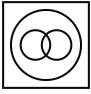3)4)QID : 217 - In the following question, select the related word from the given alternatives.
Saw : Carpenter : : Axe : ?
1) Ironsmith
2) Woodcutter
3) Cobbler
4) None of those
QID : 218 - In the following question, select the related number from the given alternatives.
8 : 27 : : 64 : ?
1) 190
2) 144
3) 125
4) 72
QID : 219 - In the following question, select the related letters from the given alternatives.
NP : HJ : : SY : ?
1) NT
2) MS
3) MT
4) NS
QID : 220 - In the following question, select the word which cannot be formed using the letters of the given word.
BILLIONAIRE
1) RANT
2) BONE
3) RAIN
4) LINE
QID : 221 - The ratio of present ages of Raman and Lamba is 7 : 5. After 6 years age of Raman will be 48 years. What is the present age (in years) of Lamba?
1) 30
2) 25
3) 35
4) 40
QID : 222 - Weight of Sona is four times that of Mona. Weight of Mona is 2.5 times of Hona. Weight of Hona is 1.5 times of Dhona. Weight of Dhona is 2.5 times of Rona. Who is the heaviest?
1) Mona
2) Sona
3) Hona
4) Dhona
QID : 223 - In the following question below are given some statements followed by some conclusions. Taking the given statements to be true even if they seem to be at variance from commonly known facts, read all the conclusions and then decide which of the given conclusion logically follows the given statements.
Statements:
I. All books are pens.
II. All pens are tables.
Conclusions:
I. Some pens are tables.
II. All tables are books.
Options:
1) Only conclusion (I) follows.
2) Only conclusion (II) follows.
3) Both conclusion follow.
4) Neither conclusion (I) nor conclusion (II) follows.
Correct Answer: Only conclusion (I) follows.
QID : 224 - In a certain code language, "PAGE" is written as "QCJI". How is "CALL"written in that code language?
1) DCOP
2) DCOQ
3) DDOP
4) DCOR